# Capability Correlations and Time¶

In the second part of the analysis we will focus on how the global capabilities change with time. This part looks to answer one main question that can be divided into other severall research questions.

• How does the research and innovation in a certain field change over time?
• Are there any chronological gaps in the research throughout the years? [...]

## 1. Data Extraction ¶

Let's start by importing all of the external libraries that will be useful during the analysis.

In :
from py2neo import Graph
import numpy as np
from pandas import DataFrame
import itertools
import matplotlib.pyplot as plt
import seaborn as sns
import json
import math
import pandas as pd
import plotly
import plotly.graph_objs as go
import qgrid
from scipy import stats, spatial
from sklearn.cluster.bicluster import SpectralBiclustering
import operator
from IPython.display import display, HTML

# connection to Neo4j
local_connection_url = "http://localhost:7474/db/data"
connection_to_graph = Graph(local_connection_url)

# plotly credentials


### 1.1. The Years ¶

Not all years in the Neo4j databse contain technological assets. For this reason, two lists will be created. A completely chronological one and a database one.

In :
# query years
years_available_q = """     MATCH (n:Asset)
WITH n.year as YEAR
RETURN YEAR, count(YEAR)
ORDER BY YEAR ASC """

# create a list with the years where records exist
years_available = DataFrame(connection_to_graph.data(years_available_q)).as_matrix()[:, 0][:-1]
years_available = [int(year) for year in years_available]

# create a pure range list
first_year = int(years_available)
last_year = int(years_available[-1])
real_years = range(first_year, last_year + 1, 1)

# give information
print 'The database list starts in {}, ends in {} and contains {} years.'.format(years_available, years_available[-1], len(years_available))
print 'The real list starts in {}, ends in {} and contains {} years.'.format(real_years, real_years[-1], len(real_years))

The database list starts in 1938, ends in 2019 and contains 38 years.
The real list starts in 1938, ends in 2019 and contains 82 years.


Now that we have all of the years available, we can start building the technological capability matrixes, with a similar process to what was previsouly done.

### 1.2. Co-occurence matrix for the years ¶

#### 1.2.1. Getting the labels ¶

We start by importing a few methods from the previous notebook.

In :
def find_index(something, in_list):
return in_list.index(something)


Let's first get all of the axis that our matrixes will take.

We start by designing two queries that will help us get all of the labels of the matrix.

The labels of the non intersecting part:

In :
q_noInter_axis = """    MATCH (a:Asset)-[:CONTAINS]->(fs:Feedstock)
MATCH (a:Asset)-[:CONTAINS]->(out:Output)
MATCH (a:Asset)-[:CONTAINS]->(pt:ProcessingTech)
RETURN fs.term, pt.term, out.term, count(a)
"""

feedstocks = np.unique(DataFrame(connection_to_graph.data(q_noInter_axis)).as_matrix()[:, 1]).tolist()
proc_tech = np.unique(DataFrame(connection_to_graph.data(q_noInter_axis)).as_matrix()[:, 2]).tolist()
output = np.unique(DataFrame(connection_to_graph.data(q_noInter_axis)).as_matrix()[:, 3]).tolist()

axis_names = feedstocks + proc_tech + output
print 'The axis list has {} terms.'.format(len(axis_names))

The axis list has 289 terms.


The labels of the intersecting part:

In :
q_Inter_axis = """  MATCH (a:Asset)-[:CONTAINS]->(fs:{})
MATCH (a:Asset)-[:CONTAINS]->(t:{})
WHERE fs<>t
RETURN fs.term, t.term, count(a)
"""
process_variables = ['Feedstock', 'Output', 'ProcessingTech']

# Extra labels that only appear in non-intersection queries
for category in process_variables:
data_no_intersections = DataFrame(connection_to_graph.data(q_Inter_axis.format(category, category))).as_matrix()
for column_number in range(1,3):
column = data_no_intersections[:, column_number]
for name in column:
if name not in axis_names:
axis_names.append(name)

print 'The axis list has {} terms.'.format(len(axis_names))

The axis list has 342 terms.


#### 1.2.2. Function ¶

We start by creating a function that given a certain year, returns the year's capability matrix.

In :
def get_year_matrix(year, normalization=True):

# define queries
q1 = """   MATCH (a:Asset)-[:CONTAINS]->(fs:Feedstock)
MATCH (a:Asset)-[:CONTAINS]->(out:Output)
MATCH (a:Asset)-[:CONTAINS]->(pt:ProcessingTech)
WHERE a.year = "{}"
RETURN fs.term, pt.term, out.term, count(a)
""".format(year)

process_variables = ['Feedstock', 'Output', 'ProcessingTech']

q2 = """   MATCH (a:Asset)-[:CONTAINS]->(fs:{})
MATCH (a:Asset)-[:CONTAINS]->(t:{})
WHERE fs<>t AND a.year = "{}"
RETURN fs.term, t.term, count(a)
"""
q3 = """
MATCH (n:Asset)
WITH n.year as YEAR
RETURN YEAR, count(YEAR)
ORDER BY YEAR ASC
"""

raw_data_q3 = DataFrame(connection_to_graph.data(q3)).as_matrix()
index_of_year = list(raw_data_q3[:, 0]).index('{}'.format(year))
total_documents = raw_data_q3[index_of_year, 1]

# get data
data_q1 = DataFrame(connection_to_graph.data(q1)).as_matrix()

# create matrix
year_matrix = np.zeros([len(axis_names), len(axis_names)])

# for no intersections data
for row in data_q1:
# the last column is the frequency (count)
frequency = row
indexes = [find_index(element, axis_names) for element in row[1::]]
# add frequency value to matrix position not inter
for pair in itertools.combinations(indexes, 2):
year_matrix[pair, pair] += frequency
year_matrix[pair, pair] += frequency

# for intersecting data
for category in process_variables:
process_data = DataFrame(connection_to_graph.data(q2.format(category, category, year))).as_matrix()
for row in process_data:
frequency = row
indexes = [find_index(element, axis_names) for element in row[1::]]
# add frequency value to matrix position inter
for pair in itertools.combinations(indexes, 2):
year_matrix[pair, pair] += frequency / 2 # Divided by two because query not optimized
year_matrix[pair, pair] += frequency / 2 # Divided by two because query not optimized

# normalize
norm_year_matrix = year_matrix  / total_documents

# dynamic return
if normalization == True:
return norm_year_matrix
else:
return year_matrix


We finally test our function with the year 2016.

In :
year = 2017
print 'The matrix from {} has shape {} a max value of {}, a min value of {} and a mean of {}.'.format(year, get_year_matrix(year).shape, np.amax(get_year_matrix(year)), np.amin(get_year_matrix(year)), np.mean(get_year_matrix(year)))

The matrix from 2017 has shape (342, 342) a max value of 0.229850746269, a min value of 0.0 and a mean of 0.000223745334066.

In :
## call functions
colors = 'BuPu_r'
year_in_focus = 2016
# create a subplot
plt.subplots(2,1,figsize=(17,17))

# first heatmap
plt.subplot(121)
sns.heatmap(get_year_matrix(year_in_focus, normalization=False) , cmap=colors,  cbar=None, square=True, xticklabels=False, yticklabels=False)
plt.title('Capability Matrix Absolute: {}'.format(year_in_focus))

# second heatmap
plt.subplot(122)
sns.heatmap(get_year_matrix(year_in_focus, normalization=True) , cmap=colors, cbar=None, square=True, xticklabels=False, yticklabels=False)
plt.title('Capability Matrix Normalized: {}'.format(year_in_focus))
plt.show()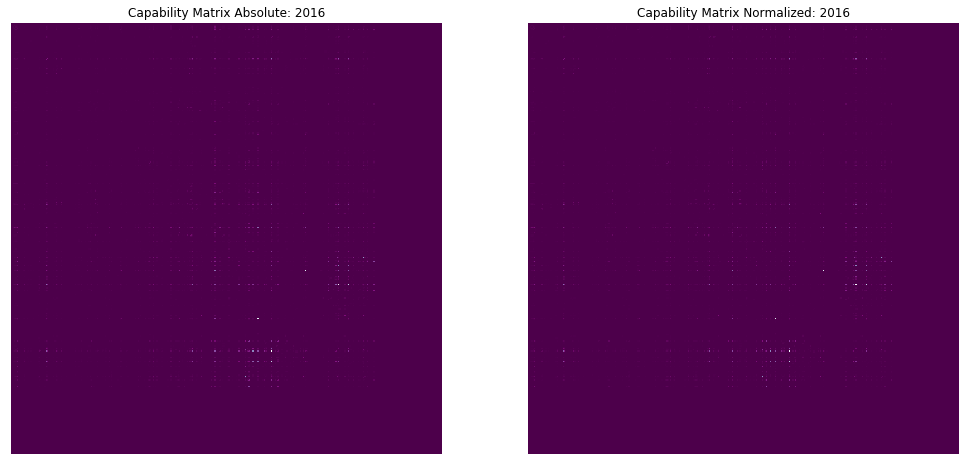## 2. Analysis ¶

In order to analyse the correlation of the years between themselves, we will need to transform each year matrix into a list. Since the matrix is symmetrical, we will only need the upper triangle. For programming reasons, we have designed our own upper triangulization matrix.

In :
def get_list_from(matrix):
only_valuable = []
extension = 1
for row_number in range(matrix.shape):
only_valuable.append(matrix[row_number, extension:matrix.shape].tolist()) # numpy functions keep 0s so I hard coded it.
extension += 1
return [element for column in only_valuable for element in column ]


### 2.1. Year Profiles ¶

Let's visualize the correlation between two years and their capability arrays.

In :
# apply functions to both countries
a_list = get_list_from(get_year_matrix(2012, normalization=True))
b_list = get_list_from(get_year_matrix(2013, normalization=True))

# create a matrix where each row is a list of a country
corelation = np.vstack((a_list, b_list))

# plot the matrix
plt.subplots(1,1,figsize=(20, 5))
plt.subplot(111)
sns.heatmap(corelation, cmap='flag_r', cbar=None, square=False, yticklabels=['2012', '2013'], xticklabels=False)
plt.yticks(rotation=0)
plt.title('Year Capability List Visualization', size=15)
plt.show()

print 'The pearson correlation index between the two years is: {} (P-value of {})'.format(stats.pearsonr(a_list, b_list), stats.pearsonr(a_list, b_list))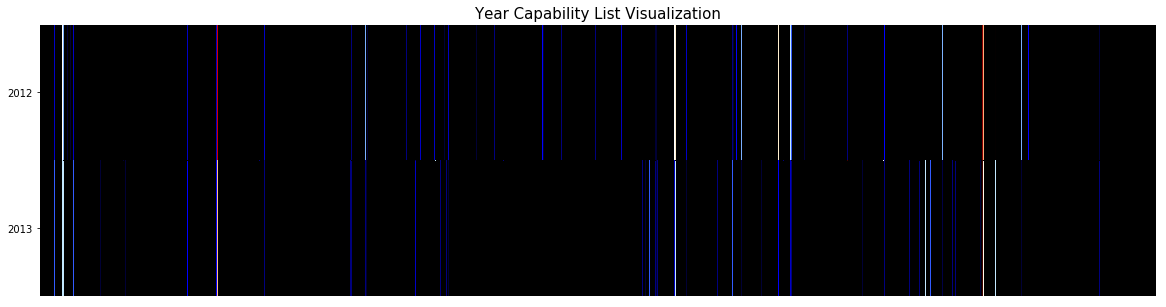The pearson correlation index between the two years is: 0.902580296089 (P-value of 0.0)


It is already apparent that these two consecutive years are highly correlated.

### 2.2. Correlation Matrix ¶

#### 2.2.1. Considerations ¶

As previously done with countries, a year correlation matrix will be built.

We first define the scope of the matrix.

In :
number_of_years = len(years_available)
years_in_matrix = years_available
years_correlation = np.zeros([number_of_years, number_of_years])
print years_in_matrix

[1938, 1975, 1980, 1981, 1983, 1985, 1986, 1988, 1989, 1990, 1992, 1993, 1994, 1995, 1996, 1997, 1998, 1999, 2000, 2001, 2002, 2003, 2004, 2005, 2006, 2007, 2008, 2009, 2010, 2011, 2012, 2013, 2014, 2015, 2016, 2017, 2018, 2019]


And we build the matrix

In :
data = np.load('Data/year_capability_dict.npy').item()
for row in range(number_of_years):
print 'Processing year {} / {} ({})\r'.format(row + 1, number_of_years, years_in_matrix[row]),
year_1_list = data[years_in_matrix[row]]
for column in range(number_of_years):

year_2_list = data[years_in_matrix[column]]

years_correlation[row, column] = stats.pearsonr(year_1_list, year_2_list)



In :
plt.subplots(1,1,figsize=(9, 9))
plt.subplot(111)
sns.heatmap(years_correlation, cbar=False, square=True, yticklabels=years_in_matrix, xticklabels=years_in_matrix)
plt.title('Years Correlation Matrix: Unordered', size=13)
plt.show()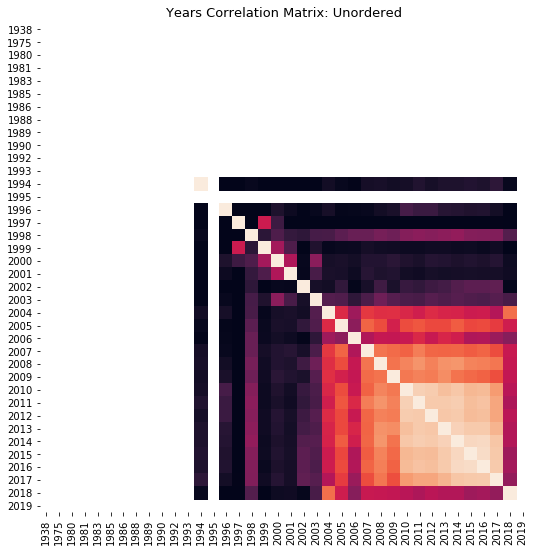There seems to be a lot of data missing.

Let's plot the amount of records in our databse over time to get a better sense on how to approach the problem.

In :
# get all of the data
data = DataFrame(connection_to_graph.data(years_available_q)).as_matrix()
raw = [int(a) for a in data[:-1, 0]]
timeline = range(min(raw), max(raw))
qtties = []

# build a timeline and number of records.
for year in timeline:
if year not in raw:
qtties.append(0)
else:
idx = find_index(str(year), list(data[:, 0]))
qtties.append(data[idx, 1])

amountOfRecords = np.column_stack((timeline, qtties))

# plot the graph
plt.style.use('seaborn-darkgrid')
plt.subplots(1,1,figsize=(16, 5))
plt.subplot(111)
plt.title("Number of assets over time")
plt.xlabel("Year")
plt.ylabel("Number of Available assets")
plt.plot(timeline, qtties)
plt.show()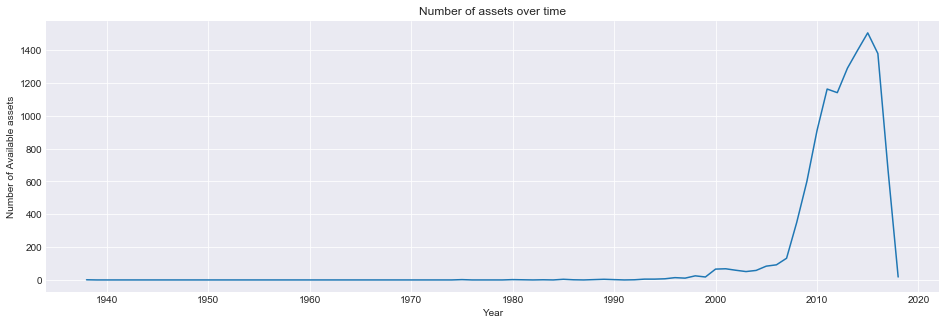#### 2.2.2. Final co-ocurrence matrix ¶

To counteract the fact that our dataset is not uniformily distributed across the years, we will only consider the last 15 years. [2004-2018]

In :
number_of_years = 22
years_in_matrix = years_available[:-1][-number_of_years:]
years_correlation = np.zeros([number_of_years, number_of_years])


We now rebuild and plot the heatmaop of correlations.

In :
data = np.load('Data/year_capability_dict.npy').item()
for row in range(number_of_years):
print 'Processing year {} / {} ({})\r'.format(row + 1, number_of_years, years_in_matrix[row]),
year_1_list = data[years_in_matrix[row]]
for column in range(number_of_years):
year_2_list = data[years_in_matrix[column]]
years_correlation[row, column] = stats.pearsonr(year_1_list, year_2_list)

plt.subplots(1,1,figsize=(8, 8))
plt.subplot(111)
sns.heatmap(years_correlation, cbar=False, square=True, yticklabels=years_in_matrix, xticklabels=years_in_matrix)
plt.title('Years Correlation Matrix: Unordered, last 15 years', size=13)
plt.show()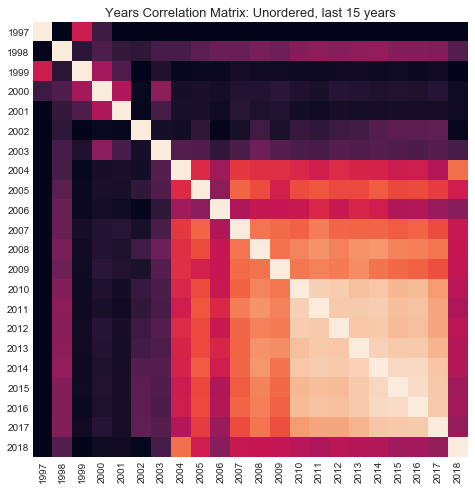#### 2.2.3. Heatmap Clustering ¶

Let us reorder the heatmap according to hierarchical clustering.

In :
# plot the clustermap
a = sns.clustermap(years_correlation,  figsize=(8, 8), xticklabels = years_in_matrix, yticklabels=years_in_matrix)
plt.show()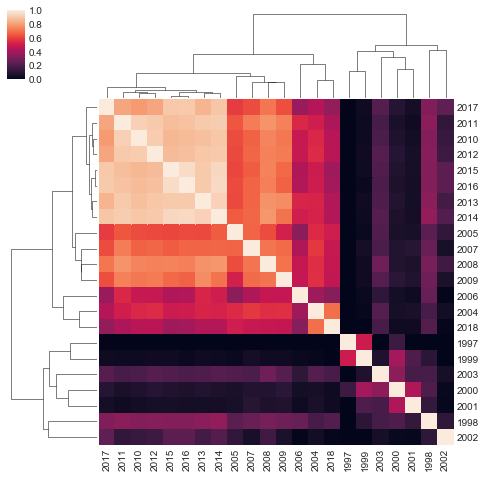### 2.3. Correlation Over Time ¶

Let us see how related is each year in our matrx with the one before it. In this way we might more easily detect discripancies.

In :
# remove first year
corr_with_pre = []

row = 1
col = 0
corr_with_pre.append(years_correlation[row, col])
row = row + 1
col = col + 1

plt.subplots(1,1,figsize=(15,7))
sns.barplot(np.arange(len(corr_with_pre)), corr_with_pre )
plt.title('Correlation of year with previous year')
plt.ylabel('Pearson Correlation Index')
plt.show()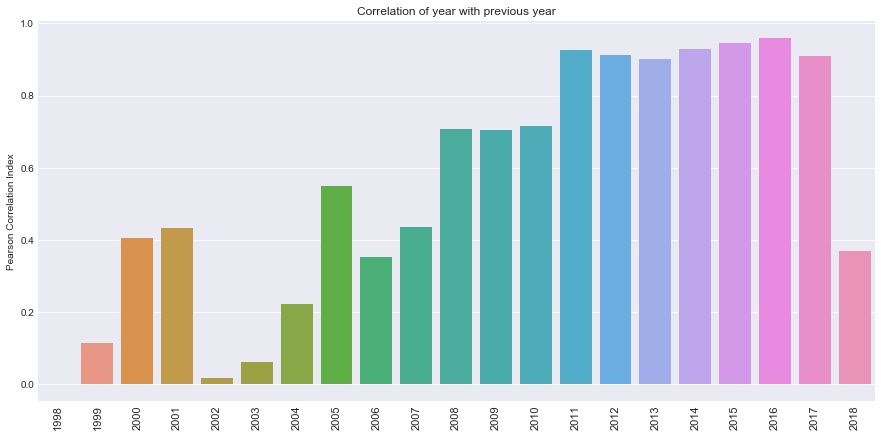Some years, such as 2006 or 2007 appear to have very low correlations with the years after. There seems to be an overall tendency of augmenting correlation with the years.

### 2.4. Research details over time ¶

The following part of the analysis wil focus on how certain process variables (Feedstocks, Processing Technologies and Outputs) evolve over time.

This can help in answering questions such as for example:

• Is the focus on a certain processing technology constant over time?
• Is this evolution correlated with other external factors?

Let's start by creating a function such as:

f(term, type of process variable) = [array with the number of records containing the term in each year]

In :
from __future__ import division
def get_records_of(startYear, endYear, term, process_type):

# make query
yearRangeQuery = """ MATCH (a:Asset)-[:CONTAINS]->(fs:{})
WHERE fs.term = "{}"
AND (toInteger(a.year)>={} AND toInteger(a.year)<={})
AND NOT a.year = "Null"
RETURN  a.year, count(a)
ORDER BY a.year   """.format(process_type, term, startYear, endYear)

# extract matrix
rawQuery = DataFrame(connection_to_graph.data(yearRangeQuery)).as_matrix()

# create matrix to store years, docs and total docs
normalTimeline = np.arange(startYear, endYear + 1)
completeMatrix = np.transpose(np.vstack((normalTimeline, normalTimeline, normalTimeline, normalTimeline)))
completeMatrix[:, 1::] = 0

# add number of docs found by query to matrix
for i in range(len(rawQuery[:, 0])):
for j in range(len(completeMatrix[:, 0])):
if int(rawQuery[i, 0]) == completeMatrix[j, 0]:
completeMatrix[j, 1] = rawQuery[i, 1]

# add total number of docs in that year to matrix
for i in range(len(completeMatrix[:, 0])):
for j in range(len(amountOfRecords[:, 0])):
if completeMatrix[i, 0] == amountOfRecords[j, 0]:
completeMatrix[i, 2] = amountOfRecords[j, 1]

# create a list of the normalized results
normalizedRecords = []
for i in range(len(completeMatrix[:, 0])):
if completeMatrix[i, 2] != 0:
normalizedRecords.append(float(completeMatrix[i, 1])/float(completeMatrix[i, 2]))
else:
normalizedRecords.append(0)

result = {}
result['range']  = completeMatrix[:, 0].tolist()
result['nominal'] = completeMatrix[:, 1].tolist()
result['total'] = completeMatrix[:, 2].tolist()
result['normalized'] = normalizedRecords

return result


Now that the function is built, we can plot virtually any evolution.

#### 2.4.1. Outputs ¶

Let us see the evolution of records of biogas Vs. ethanol as an example.

In :
listOfOutputs = ['biogas', 'ethanol', 'biodiesel']
start_year = 1990
end_year = 2017

# plot the graph
plt.style.use('seaborn-darkgrid')
plt.subplots(1,1,figsize=(16, 5))
plt.subplot(111)
plt.title("Evolution of Records with focus on Output")
plt.xlabel("Year")
plt.ylabel("Normalized Quantity")

for name in listOfOutputs:
nameData = get_records_of(start_year,end_year,name, 'Output')
plt.plot(nameData['range'], nameData['normalized'], label=name)

plt.legend()
plt.show()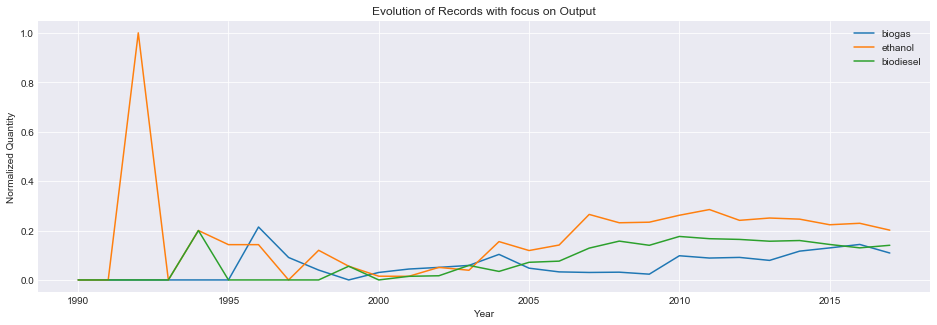#### 2.4.2. Processing technologies ¶

Let us develop the same procedure for some processign technologies.

In :
listOfProcTech = ['fermentation','enzymatic hydrolysis','hydrolysis' ]
start_year = 1990
end_year = 2017

# plot the graph
plt.style.use('seaborn-darkgrid')
plt.subplots(1,1,figsize=(16, 5))
plt.subplot(111)
plt.title("Evolution of Records with focus on Processing Technologies")
plt.xlabel("Year")
plt.ylabel("Normalized Quantity")

for name in listOfProcTech:
nameData = get_records_of(start_year,end_year,name, 'ProcessingTech')
plt.plot(nameData['range'], nameData['normalized'], label=name)

plt.legend()
plt.show()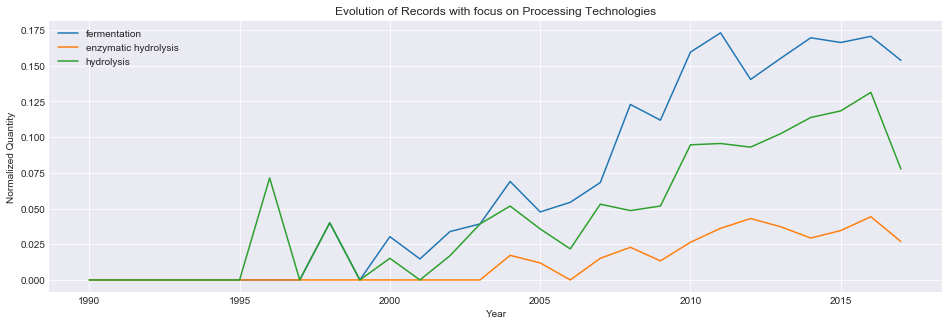#### 2.4.3. Feedstock ¶

Let us develop the same procedure for feedstock.

In :
listOfFeed = ['sugar','wood','paper', 'algae', 'waste']
start_year = 1990
end_year = 2017

# plot the graph
plt.style.use('seaborn-darkgrid')
plt.subplots(1,1,figsize=(16, 5))
plt.subplot(111)
plt.title("Evolution of Records with focus on Feedstocks")
plt.xlabel("Year")
plt.ylabel("Normalized Quantity")

for name in listOfFeed:
nameData = get_records_of(start_year,end_year,name, 'Feedstock')
plt.plot(nameData['range'], nameData['normalized'], label=name)

plt.legend()
plt.show()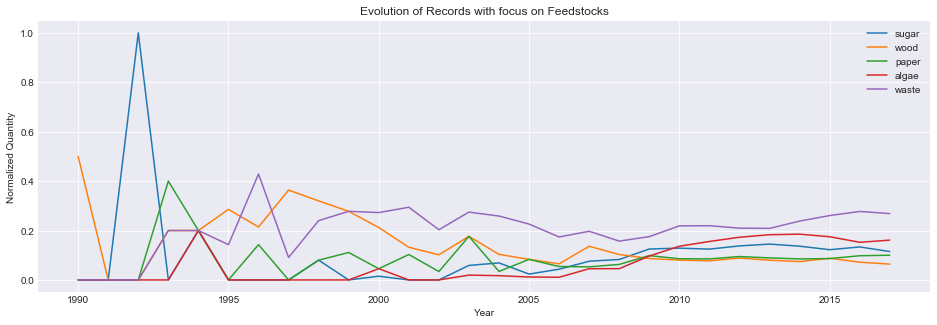### 2.5. Contextual Relationships ¶

#### 2.5.1. US Regular Conventional Gas Price ¶

We start by comparing the evolution of the outputs above studied with the average oil price per gallon found in the following website.

We import the data, and convert monthly prices to yearly averages with the bellow code.

In :
# get price per gallon in US dollars
gallon = []
oil_years = list(set([int(e[0:4]) for e in oil_data[:, 0]]))[:-1]
for year in oil_years:
months = 0
for row in oil_data:
if str(year) in row:
months += 1
gallon.append(average)

# get price per barrel data

oil_index = {'gallon':gallon, 'barrel':barrel}


Relationship Over Time

Let us visualize how the evolution of the price of gas relates to the normalized quantity of assets over time, in a chronological graph.

In :
# define subplots
fig, ax1 = plt.subplots(figsize=(15,7))
listOfOutputs = ['biogas', 'bioplastic', 'butanol']
colors = ['b', 'y', 'g']
start_year = 1990
end_year = 2017
price_type = 'barrel'

# first axis

for position, outputName in enumerate(listOfOutputs):
nameData = get_records_of(start_year, end_year, outputName, 'Output')
ax1.plot(nameData['range'], nameData['normalized'], label=outputName, color=colors[position], ls='--', alpha=0.5)

ax1.set_xlabel('Years')
ax1.set_ylabel('Number of relative records')
ax1.tick_params('y')
ax1.set_title('Oil Price Vs. Asset Quantity')
ax1.legend(loc=2, frameon=True)
ax1.grid(False)

# second axis
ax2 = ax1.twinx()
ax2.plot(oil_years,oil_index[price_type], color='r', label='Oil Price')
ax2.set_ylabel('Price of {} of oil $US'.format(price_type), color='r') ax2.tick_params('y', colors='r') ax2.legend(loc=1, frameon=True) # expose plt.show()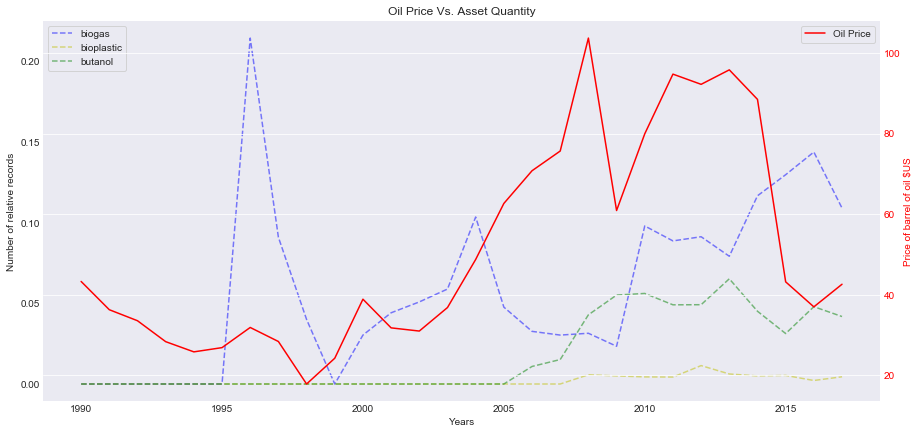Scatter Visualization To study this relationship in a more in depth fashio we create a process that given a certain term gives us the relationship with the price of gas. In : outPutToCompare = 'butanol' typeOfProcessVariable = 'Output' price_type = 'gallon' data = get_records_of(1990, 2017, outPutToCompare, typeOfProcessVariable)['normalized'] fig, ax1 = plt.subplots(figsize=(15,7)) sns.regplot(np.asarray(oil_index[price_type]), np.asarray(data) ,fit_reg=True, marker="+", color = 'g') plt.title('Gas price relation with quantity of Assets: {}'.format(outPutToCompare)) plt.xlabel('Price of {} of oil in US$ in Year'.format(price_type))
plt.ylabel('Quantity of Asset {} in Year'.format(outPutToCompare))

plt.show()

correlationIndexes =  stats.pearsonr(np.asarray(oil_index[price_type]), np.asarray(get_records_of(1990, 2017, outPutToCompare, 'Output')['normalized']))
print 'Pearson Correlation Index: ', correlationIndexes
print 'P-value: ', correlationIndexes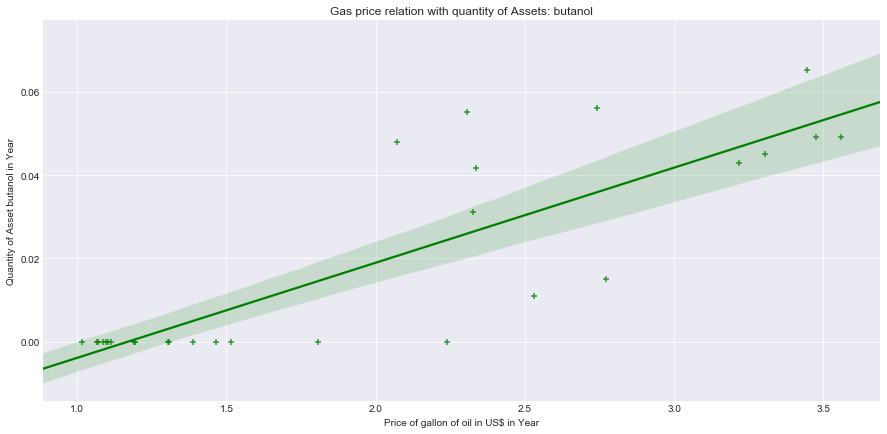Pearson Correlation Index:  0.8445465111603638
P-value:  1.6031894575347735e-08


In the above graph each datapoint corresponds to a year.

Ranking of Most Related Outputs

In :
term_names_query = """  MATCH (a:Asset)-[:CONTAINS]->(fs:Output)
WHERE (toInteger(a.year)>=1990 AND toInteger(a.year)<=2017)
AND NOT a.year = "Null"
RETURN  fs.term, count(a)
ORDER BY count(a) DESC"""
oil_type = 'gallon'
term_names = list(DataFrame(connection_to_graph.data(term_names_query)).as_matrix()[:, 1].tolist())
correlations = []
p_values = []
for term in term_names:
data = get_records_of(1990, 2017, term, 'Output')['normalized']
correlations.append(stats.pearsonr(data, oil_index[oil_type]))
p_values.append(stats.pearsonr(data, oil_index[oil_type]))

oilDataFrame = pd.DataFrame(
{'Output Name': term_names,
'Pearson Correlation Index': correlations,
'P-value': p_values
})
oilDataFrame = oilDataFrame.sort_values('Pearson Correlation Index', ascending=False)

print 'The relationship between relative number of documents and price of oil over time:'
top = 10

print 'TOP {}:'.format(top)
display(oilDataFrame[:top])

The relationship between relative number of documents and price of oil over time:
TOP 10:

Output Name P-value Pearson Correlation Index
7 butanol 1.603189e-08 0.844547
19 bioplastic 2.463734e-07 0.804599
1 biodiesel 7.978637e-07 0.784034
21 fatty acid ethyl ester 1.427601e-06 0.772960
3 bioethanol 2.862862e-05 0.704439
9 syng 3.649295e-05 0.697899
15 biobutanol 5.140385e-05 0.688369
8 cellulosic ethanol 1.301892e-04 0.660616
14 biopolymers 3.263515e-04 0.630094

Negative Correlations

In :
term_names_query = """  MATCH (a:Asset)-[:CONTAINS]->(fs:Output)
WHERE (toInteger(a.year)>=1990 AND toInteger(a.year)<=2017)
AND NOT a.year = "Null"
RETURN  fs.term, count(a)
ORDER BY count(a) DESC"""
oil_type = 'gallon'
term_names = list(DataFrame(connection_to_graph.data(term_names_query)).as_matrix()[:, 1].tolist())
correlations = []
p_values = []
for term in term_names:
data = get_records_of(1990, 2017, term, 'Output')['normalized']
correlations.append(stats.pearsonr(data, oil_index[oil_type]))
p_values.append(stats.pearsonr(data, oil_index[oil_type]))

oilDataFrame = pd.DataFrame(
{'Output Name': term_names,
'Pearson Correlation Index': correlations,
'P-value': p_values
})
oilDataFrame = oilDataFrame.sort_values('Pearson Correlation Index', ascending=False)

print 'The relationship between relative number of documents and price of oil over time:'
bottom = -10

print 'BOTTOM {}:'.format(bottom)
display(oilDataFrame[bottom:])

The relationship between relative number of documents and price of oil over time:
BOTTOM -10:

Output Name P-value Pearson Correlation Index
16 naphtha 0.677733 0.082145
44 biodiesel blending 0.683315 0.080645
45 ethanol blending 0.683315 0.080645
18 renewable diesel 0.716673 0.071767
11 renewable fuel 0.944557 0.013770
17 succinic acid 0.956893 0.010703
40 rdif 0.629618 -0.095276
34 electricity from biomass 0.456174 -0.146748
5 gasoline 0.371514 -0.175570
10 pellets 0.268436 -0.216520

#### 2.5.2. Sugar Cost ¶

In this part we will make the same analysis but taking an example of a feedstock: sugar.

Data was obtained here.

We start by importing the data.

In :
sugar_data = pd.read_csv('Data/Sugar_Price.csv', delimiter=';', header=None).as_matrix()
sugar = {}
sugar['years'] = [int(e) for e in sugar_data[:, 0]]
sugar['nominal'] = [e for e in sugar_data[:, 1]]
sugar['real'] =  [e for e in sugar_data[:, 2]]


Relationship Over Time

Let us see the evolution of Sugar prices side by side with the evolution of certain feedstocks in our database.

In :
# define subplots
fig, ax1 = plt.subplots(figsize=(15,7))
feedstock_list = ['sugar', 'wood', 'sugarcane', 'sugar beet', 'cellulosic sugars']
colors = ['gold', 'mediumblue', 'm', 'green', 'k']

start_year = 1990
end_year = 2017
sugar_price_type = 'real'

# first axis
for position,feedstock in enumerate(feedstock_list):
data = get_records_of(start_year, end_year, feedstock, 'Feedstock')
ax1.plot(data['range'], data['normalized'], label=feedstock, ls='--', color=colors[position])

ax1.set_xlabel('Years')
ax1.set_ylabel('Relative number of records')
ax1.tick_params('y')
ax1.set_title('Sugar Prices Vs. Asset Quantity')
ax1.legend(loc=3, frameon=True)
ax1.grid(False)

# second axis
ax2 = ax1.twinx()
ax2.plot(sugar['years'], sugar[sugar_price_type], color='r', label='Sugar Price', ls='-')
ax2.set_ylabel('Price per kilo of sugar in $US (inflation adjusted)', color='r') ax2.tick_params('y', colors='r') ax2.legend(loc=1, frameon=True) # expose plt.show()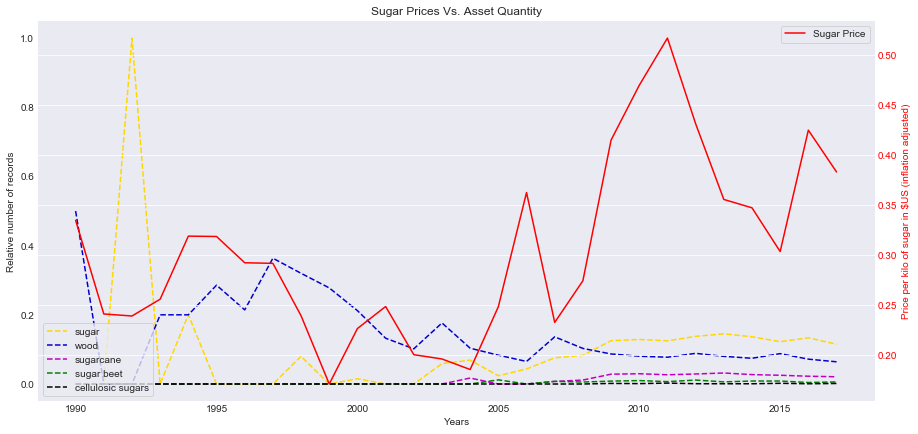Scatter Example Let us see a scatter plot where each point is a year and the x and y axis correpond to the price of sugar and quantity of assets respectively. In : outPutToCompare = 'sugarcane' typeOfProcessVariable = 'Feedstock' price_type = 'real' data = get_records_of(1990, 2017, outPutToCompare, typeOfProcessVariable)['normalized'] fig, ax1 = plt.subplots(figsize=(15,7)) sns.regplot(np.asarray(sugar[price_type]), np.asarray(data) ,fit_reg=True, marker="+", color = 'b') plt.title('Sugar price relation with quantity of Assets: {}'.format(outPutToCompare)) plt.xlabel('Price of sugar US$ per kilo in Year ({})'.format(price_type))
plt.ylabel('Quantity of Asset {} in Year'.format(outPutToCompare))

plt.show()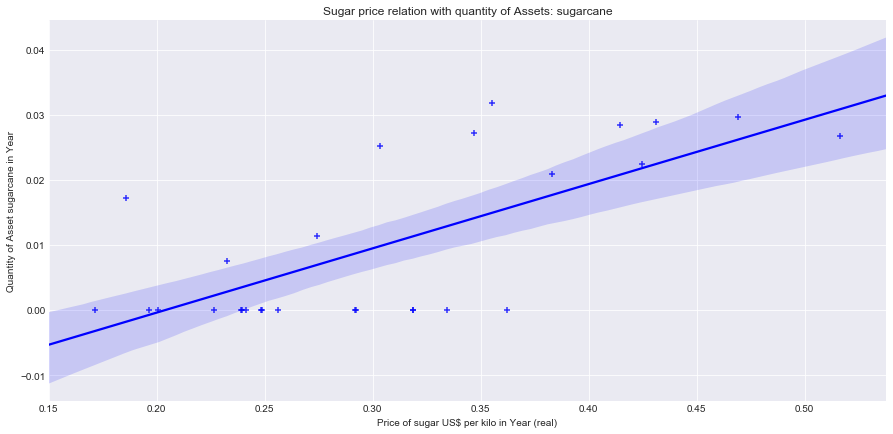Most Related Feedstocks

Which are the feedstocks who are more related to the price of sugar per kilo in what regards the number of records?

In :
term_names_query = """  MATCH (a:Asset)-[:CONTAINS]->(fs:Feedstock)
WHERE (toInteger(a.year)>=1990 AND toInteger(a.year)<=2017)
AND NOT a.year = "Null"
RETURN  fs.term, count(a)
ORDER BY count(a) DESC"""
price_type = 'nominal'
term_names = list(DataFrame(connection_to_graph.data(term_names_query)).as_matrix()[:, 1].tolist())
correlations = []
p_values = []
for term in term_names:
data = get_records_of(1990, 2017, term, 'Feedstock')['normalized']
correlations.append(stats.pearsonr(data, sugar[price_type]))
p_values.append(stats.pearsonr(data, sugar[price_type]))

sugarDataframe = pd.DataFrame(
{'Feedstock Name': term_names,
'Pearson Correlation Index': correlations,
'P-value': p_values
})
sugarDataframe = sugarDataframe.sort_values('Pearson Correlation Index', ascending=False)

print 'The relationship between relative number of documents and price per kilo of sugar:'
top = 10

print 'TOP {}:'.format(top)
display(sugarDataframe[:top])

The relationship between relative number of documents and price per kilo of sugar:
TOP 10:

Feedstock Name P-value Pearson Correlation Index
26 sugarcane 6.074365e-07 0.789014
107 cellulosic sugars 1.263220e-06 0.775341
43 jatropha 1.521222e-06 0.771713
34 sorghum 3.083429e-06 0.757299
64 dry biomass 3.454736e-06 0.754884
75 beets 4.105286e-06 0.751165
99 dedicated energy crops 6.915371e-06 0.739525
1 algae 1.103076e-05 0.728562
100 hybrid poplar 1.490683e-05 0.721206
25 soy 2.631077e-05 0.706675

Negative Correlations

In :
term_names_query = """  MATCH (a:Asset)-[:CONTAINS]->(fs:Feedstock)
WHERE (toInteger(a.year)>=1990 AND toInteger(a.year)<=2017)
AND NOT a.year = "Null"
RETURN  fs.term, count(a)
ORDER BY count(a) DESC"""
price_type = 'nominal'
term_names = list(DataFrame(connection_to_graph.data(term_names_query)).as_matrix()[:, 1].tolist())
correlations = []
p_values = []
for term in term_names:
data = get_records_of(1990, 2017, term, 'Feedstock')['normalized']
correlations.append(stats.pearsonr(data, sugar[price_type]))
p_values.append(stats.pearsonr(data, sugar[price_type]))

sugarDataframe = pd.DataFrame(
{'Feedstock Name': term_names,
'Pearson Correlation Index': correlations,
'P-value': p_values
})
sugarDataframe = sugarDataframe.sort_values('Pearson Correlation Index', ascending=False)

print 'The relationship between relative number of documents and price per kilo of sugar:'
bottom = -10

print 'Bottom {}:'.format(bottom * -1)
display(sugarDataframe[bottom:])

The relationship between relative number of documents and price per kilo of sugar:
Bottom 10:

Feedstock Name P-value Pearson Correlation Index
93 wood fuel 0.336664 -0.188530
72 waste oil 0.329527 -0.191282
136 particle board 0.289545 -0.207425
156 durum 0.279399 -0.211741
178 citrus residues 0.260452 -0.220080
123 beef tallow 0.240798 -0.229158
53 sawdust 0.223542 -0.237542
138 trap grease 0.212719 -0.243025
79 wood waste 0.210637 -0.244102
5 wood 0.137684 -0.287685

NON SERIES TIME ANALYSIS IS A LIMITATION.

### 2.6. In depth year comparison ¶

In this part of the analysis the goal is two understand what exact capabilities differ from year to year. More exactly, how does one particular capability evolve over the course of two or more years.

For example, if in year X1, Y1% of the assets related to sugar, what is the percentage Y2% in year X2?

#### 2.6.1. Visualizing the differences ¶

Let us visualize two different years side by side.

In :
## call functions
first_year = 2017
second_year = 2010
colors = 'BuPu_r'
fst_year_matrix = get_year_matrix(first_year, normalization=False)
scnd_year_matrix = get_year_matrix(second_year, normalization=False)

# create a subplot
plt.subplots(2,1,figsize=(17,17))

# first heatmap
plt.subplot(121)
sns.heatmap(fst_year_matrix, cmap=colors,  cbar=None, square=True, xticklabels=False, yticklabels=False)
plt.title('Capability Matrix: {}'.format(first_year))

# second heatmap
plt.subplot(122)
sns.heatmap(scnd_year_matrix, cmap=colors, cbar=None, square=True, xticklabels=False, yticklabels=False)
plt.title('Capability Matrix: {}'.format(second_year))
plt.show()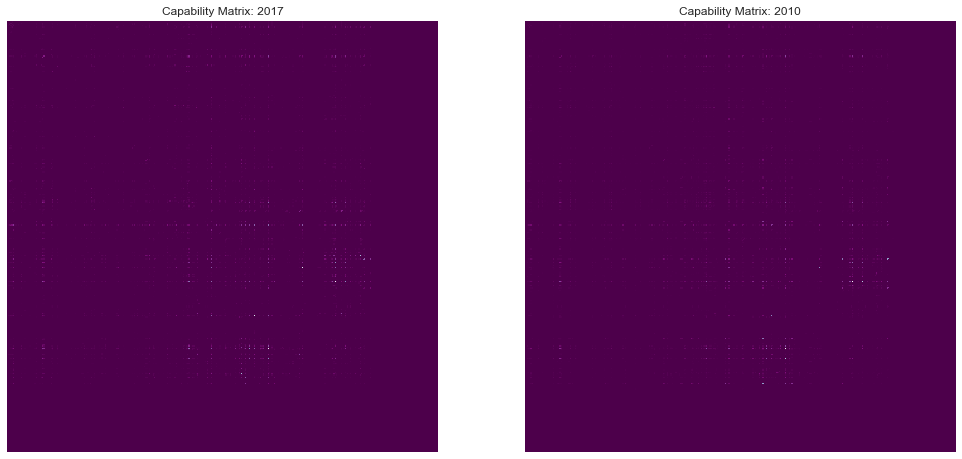Due to the very high number of rows, visualization is rather hard.

The next step is to create a matrix of absolute diferences between the two examples, for this, we start by subtracting them:

In :
cap_diff = np.absolute(fst_year_matrix - scnd_year_matrix)


And we plot these differences.

In :
plt.subplots(1,1,figsize=(13, 13))
plt.subplot(111)
sns.heatmap(cap_diff, cmap=colors, square=True, yticklabels=False, xticklabels=False)
plt.title('Differences between {} and {}: Normalized Differences'.format(first_year, second_year), size=13)
plt.show()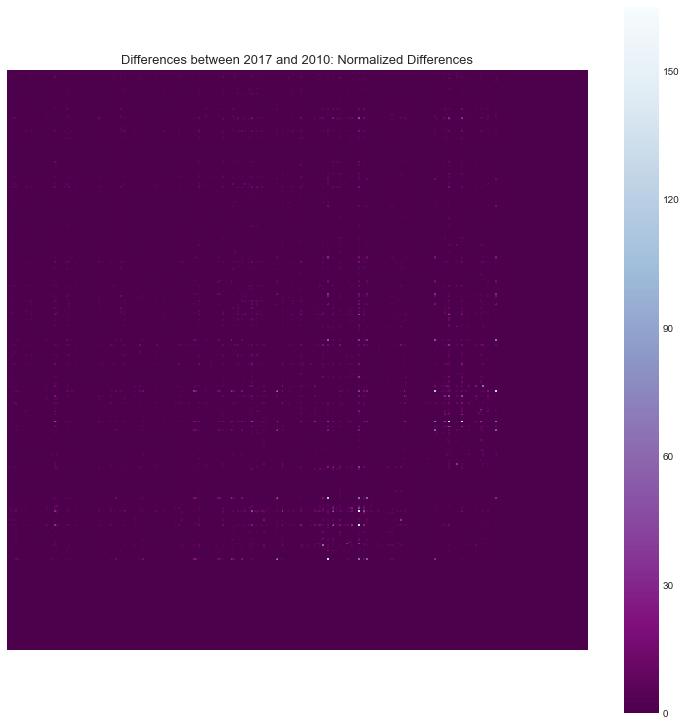There seem to be some areas where differences clearly exist. Let us investigate these areas in a more in depth fashion.

#### 2.6.2. Understanding the differences ¶

Let's understand what exact capability pairs are the most 'popular' in each year.

We start by creating a function that returns given a year X, the most popular capability pairs of that year as absolute numbers and percentage of total documents.

In :
def get_top_hits(yearMatrix, year):
"""
The function prints the top occurences if fed a matrix of occurences, it also prints other types of valuable info.
WARNING: Percentages are shown as 0 to 1.
"""

# list where all the values and indexes of matrix are stored
top = 10
values = []
indexes = []
no_duplicates = np.triu(yearMatrix, 1)
total_documents = np.sum(no_duplicates)
matrix_axis_names = axis_names

# loop through the matrix
for row_n in range(yearMatrix.shape):
for col_n in range(yearMatrix.shape):
values.append(no_duplicates[row_n, col_n])
indexes.append((row_n, col_n))

# order the indexes and get the top
Z = [indexes for _,indexes in sorted(zip(values,indexes))]
extremes = Z[-top :]

# create dataframe
term_Dataframe = pd.DataFrame(
{'First Term': [matrix_axis_names[e] for e in extremes],
'Second Term': [matrix_axis_names[e] for e in extremes],
'Number of Documents': [int(no_duplicates[e, e]) for e in extremes],
'Percentage' : [no_duplicates[e, e] / float(total_documents) for e in extremes],
})

# prepare dataframe
term_Dataframe = term_Dataframe[['First Term', 'Second Term','Number of Documents', 'Percentage']]
term_Dataframe = term_Dataframe.sort_values('Number of Documents', ascending=False)

# print everything
print 'The top hits for the {} matrix: '.format(year)
display(HTML(term_Dataframe.to_html(index=False)))

print 'The total number of documents is {}.'.format(int(total_documents))
print 'Note: Percentages are as 0-1 in this table. '


Let us use this function to try to understand each year.

In :
get_top_hits(fst_year_matrix, first_year)

The top hits for the 2017 matrix:

First Term Second Term Number of Documents Percentage
ethanol fermentation 154 0.017566
biogas anaerobic digestion 137 0.015627
bio-oil pyrolysis 101 0.011520
bioethanol fermentation 76 0.008669
ethanol hydrolysis 76 0.008669
sugar ethanol 60 0.006844
waste ethanol 58 0.006616
sugar fermentation 57 0.006502
waste biogas 53 0.006045
biogas fermentation 53 0.006045
The total number of documents is 8767.
Note: Percentages are as 0-1 in this table.

In :
get_top_hits(scnd_year_matrix, second_year)

The top hits for the 2010 matrix:

First Term Second Term Number of Documents Percentage
ethanol fermentation 319 0.022040
ethanol hydrolysis 225 0.015545
biodiesel transesterification 168 0.011607
biogas anaerobic digestion 152 0.010502
biodiesel catalysis 131 0.009051
bioethanol fermentation 120 0.008291
sugar ethanol 106 0.007323
sugar fermentation 102 0.007047
bioethanol hydrolysis 95 0.006563
ethanol enzymatic hydrolysis 85 0.005873
The total number of documents is 14474.
Note: Percentages are as 0-1 in this table.


We can make two observations:

• These two particular years have generally the same term pairs in their top table.
• However, the percentages can differ greatly.

Note: There is a high difference in number of documents.

Let us now create a side by side comparison.

In :
# list where all the values and indexes of matrix are stored
frst_perc = fst_year_matrix / np.sum(np.triu(fst_year_matrix, 1)) # half only
scnd_perc = scnd_year_matrix / np.sum(np.triu(scnd_year_matrix, 1))
differences = frst_perc - scnd_perc
differences = np.absolute(differences)
values = []
indexes = []
no_duplicates = np.triu(differences, 1)
matrix_axis_names = axis_names

top = 20

# loop through the matrix
for row_n in range(differences.shape):
for col_n in range(differences.shape):
values.append(no_duplicates[row_n, col_n])
indexes.append((row_n, col_n))

# print the table
Z = [indexes for _,indexes in sorted(zip(values,indexes))]
extremes = list(reversed(Z[-top:]))

term_Dataframe = pd.DataFrame(
{'First Term': [matrix_axis_names[e] for e in extremes],
'Second Term': [matrix_axis_names[e] for e in extremes],
'{} Percentage'.format(first_year): [frst_perc[e, e] for e in extremes],
'{} Percentage'.format(second_year): [scnd_perc[e, e] for e in extremes],
'Difference in %': [no_duplicates[e, e] for e in extremes]
})

term_Dataframe = term_Dataframe[['First Term', 'Second Term', '{} Percentage'.format(first_year), '{} Percentage'.format(second_year), 'Difference in %']]

display(HTML(term_Dataframe.to_html(index=False)))
print 'Percentages are as 0-1 in this table for easy viz.'

First Term Second Term 2017 Percentage 2010 Percentage Difference in %
bio-oil pyrolysis 0.011520 0.002625 0.008895
biodiesel transesterification 0.003878 0.011607 0.007729
biodiesel catalysis 0.001825 0.009051 0.007226
ethanol hydrolysis 0.008669 0.015545 0.006876
biogas anaerobic digestion 0.015627 0.010502 0.005125
ethanol anaerobic digestion 0.005133 0.000553 0.004580
ethanol fermentation 0.017566 0.022040 0.004474
vegetable oil transesterification 0.000570 0.005044 0.004473
syng gasification 0.004563 0.000345 0.004217
ethanol catalysis 0.000798 0.004974 0.004176
ethanol transesterification 0.001825 0.005596 0.003771
methanol catalysis 0.000114 0.003869 0.003755
biodiesel solvents 0.005703 0.002073 0.003631
waste anaerobic digestion 0.005817 0.002556 0.003261
gasoline fermentation 0.000342 0.003454 0.003112
vegetable oil catalysis 0.000913 0.004007 0.003095
methanol transesterification 0.001711 0.004629 0.002918
bioethanol anaerobic digestion 0.002966 0.000069 0.002897
waste bio-oil 0.003764 0.000898 0.002866
vegetable oil biodiesel 0.002509 0.005320 0.002810
Percentages are as 0-1 in this table for easy viz.


With this visualization we can easily compare the term pairs and see their evolution over the course of the years.

In [ ]:


In [ ]: